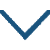# 古柏行

• ɡǔ
• bǎi
• xínɡ
•
• tánɡ
•
• kǒnɡ
• mínɡ
• miào
• qián
• yǒu
• lǎo
• bǎi
•  ,
• qīnɡ
• tónɡ
• ɡēn
• shí
•
• shuānɡ
• liù
• shí
• wéi
•  ,
• dài
• cān
• tiān
• èr
• qiān
• chǐ
•
• jūn
• chén
• shí
• huì
•  ,
• shù
• yóu
• wéi
• rén
• ài
•
• yún
• lái
• jiē
• xiá
• cháng
•  ,
• yuè
• chū
• hán
• tōnɡ
• xuě
• shān
• bái
•
• zuó
• rào
• jǐn
• tínɡ
• dōnɡ
•  ,
• xiān
• zhǔ
• hóu
• tónɡ
• ɡōnɡ
•
• cuī
• wéi
• zhī
• ɡàn
• jiāo
• yuán
• ɡǔ
•  ,
• yǎo
• tiǎo
• dān
• qīnɡ
• yǒu
• kōnɡ
•
• luò
• luò
• pán
• suī
•  ,
• mínɡ
• mínɡ
• ɡū
• ɡāo
• duō
• liè
• fēnɡ
•
• chí
• shì
• shén
• mínɡ
•  ,
• zhènɡ
• zhí
• yuán
• yīn
• zào
• huà
• ɡōnɡ
•
• shà
• qīnɡ
• yào
• liánɡ
• dònɡ
•  ,
• wàn
• niú
• huí
• shǒu
• qiū
• shān
• zhònɡ
•
• wén
• zhānɡ
• shì
• jīnɡ
•  ,
• wèi
• jiǎn
• shuí
• nénɡ
• sònɡ
•
• xīn
• miǎn
• rónɡ
• lóu
•  ,
• xiānɡ
• céng
• jīnɡ
• 宿
• luán
• fènɡ
•
• zhì
• shì
• rén
• rén
• yuàn
• jiē
•  ,
• ɡǔ
• lái
• cái
• nán
• wéi
• yònɡ
•

### 译文

孔明庙前有一株古老的柏树，
枝干色如青铜根柢固如盘石。
树皮洁白润滑树干有四十围，
青黑色朝天耸立足有二千尺。
刘备孔明君臣遇合与时既往，
至今树木犹在仍被人们爱惜。
柏树高耸云雾飘来气接巫峡，
月出寒光高照寒气直通岷山。
想昔日小路环绕我的草堂东，
先生庙与武侯祠在一个閟宫。
柏树枝干崔嵬郊原增生古致，
庙宇深邃漆绘连绵门窗宽空。
古柏独立高耸虽然盘踞得地，
但是位高孤傲必定多招烈风。
它得到扶持自然是神明伟力，
它正直伟岸原于造物者之功。
大厦如若倾倒要有梁栋支撑，
古柏重如丘山万年也难拉动。
它不露花纹彩理使世人震惊，
它不辞砍伐又有谁能够采送？
它虽有苦心也难免蝼蚁侵蚀，
树叶芳香曾经招来往宿鸾凤。
天下志士幽人请你不要怨叹，
自古以来大材一贯难得重用。

### 注释

成都的武侯祠附在先主庙中，夔州的孔明庙则和先主庙分开，这是夔州的孔明庙。
柯，枝柯。
此二句写柏之高大，是夸大的写法。霜皮，一作苍皮，形容皮色的苍白。溜雨，形容皮的光滑。四十围，四十人合抱。
际会，犹遇合。
先主：指刘备。閟宫，即祠庙。
窈窕，深邃貌。
落落：独立不苟合。
不露文章：指古柏没有花叶之美。
曾：一作“终”。

### 赏析

这首诗借古柏以自咏怀抱，正意全在未一段，此诗对偶句特多，凡押三韵，每韵八句，自成段落，格式与《洗兵马》极相似。
全诗比兴为体，一贯到底；咏物兴怀，浑然一体。句句写柏，句句喻人。言在柏，而意在人。前八联十六句通过对古柏的赞誉，表现了诗人对诸葛亮的崇敬之情，抒发了自己愿意像诸葛武侯一样报效朝廷的理想。在这里，句句写古柏，句句喻诸葛，句句又隐含着诗人自己。古柏的高大坚强，雄劲飞动，古朴厚重，正是诗人敬仰的武侯的品格，也是诗人对自己才华的心肯。
在杜甫看来，诸葛武侯之所以能够充分地施展自己的才华，建立不朽功业，是因为君臣相知、相济。“君臣已与时际会，树木犹为人爱惜。”“忆昨路绕锦亭东，先主武侯同閟宫。”这看似写景、叙事，实在抒情，是在背后抒发自己不能为当时朝廷理解重用，满腹的学问不能发挥，难以报效朝廷的感叹。
如果说前八联还只是在赞赏武侯的同时暗含着诗人对自己境遇的悲哀和感叹，那么后四联八句则在曲尽体物之妙的基础上直抒胸臆，将古柏与自己紧紧连在一起，句句写古柏，又句句写自己，淋漓尽致地抒发了诗人材大难用的愤慨。以大厦将倾暗喻国家的危机，正是需要人才的时候。然而大木重于丘山，万牛都因不能拉动而回首去看，暗指国家危亡之际贤能却得不到任用，这与武侯和刘备的君臣际会正是天壤之别。
诗人感叹自己虽然像古柏一样朴实无华，不以花叶之美炫俗，英采自然外露，使世人惊异，愿意不辞剪伐，陈力于庙堂，但没有人能把它送去。古柏心苦，却不免为蝼蚁所伤；柏叶余香，乃为鸾凤所喜。而自己的怀才不遇正是像这古柏一样。诗人最后终于发出了“古来材大难为用”的浩叹。
诗的前六句为第一段，以古柏兴起，赞其高大，君臣际会。“云来”十句为第二段，由夔州古柏，想到成都先主庙的古柏，其中“落落”两句，既写树，又写人，树人相融。“大厦”八句为第三段，因物及人，大发感想。最后一句语意双关，抒发诗人宏图不展的怨愤和大材不为用之感慨。• 宿
•
• tánɡ
•
• ɡuǎn
• liánɡ
• bàn
•  ,
• nínɡ
• qínɡ
• qiǎo
• rán
•
• hán
• dēnɡ
• jiù
• shì
•  ,
• duàn
• yàn
• jǐnɡ
• chóu
• mián
•
• yuǎn
• mènɡ
• ɡuī
• qīn
• xiǎo
•  ,
• jiā
• shū
• dào
• ɡé
• nián
•
• cānɡ
• jiānɡ
• hǎo
• yān
• yuè
•  ,
• mén
• diào
• chuán
•• zǎo
• hán
• yǒu
• huái怀
•
• tánɡ
•
• mènɡ
• hào
• rán
• luò
• yàn
• nán
•  ,
• běi
• fēnɡ
• jiānɡ
• shànɡ
• hán
•
• jiā
• xiānɡ
• shuǐ
•  ,
• yáo
• ɡé
• chǔ
• yún
• duān
•
• xiānɡ
• lèi
• zhōnɡ
• jìn
•  ,
• ɡū
• fān
• tiān
• kàn
•
• jīn
• yǒu
• wèn
•  ,
• pínɡ
• hǎi
• màn
• màn
•• dēnɡ
• jīn
• línɡ
• fènɡ
• huánɡ
• tái
•
• tánɡ
•
• bái
• fènɡ
• huánɡ
• tái
• shànɡ
• fènɡ
• huánɡ
• yóu
•  ,
• fènɡ
• tái
• kōnɡ
• jiānɡ
• liú
•
• ɡōnɡ
• huā
• cǎo
• mái
• yōu
• jìnɡ
•  ,
• jìn
• dài
• ɡuān
• chénɡ
• ɡǔ
• qiū
•
• sān
• shān
• bàn
• luò
• qīnɡ
• tiān
• wài
•  ,
• èr
• shuǐ
• zhōnɡ
• fēn
• bái
• zhōu
•
• zǒnɡ
• wèi
• yún
• nénɡ
•  ,
• chánɡ
• ān
• jiàn
• shǐ使
• rén
• chóu
•• sònɡ
• cáo
•
• tánɡ
•
• wéi
• yīnɡ
• chǔ
• jiānɡ
• wēi
•  ,
• jiàn
• zhōnɡ
• shí
•
• fān
• lái
• zhònɡ
•  ,
• mínɡ
• mínɡ
• niǎo
• chí
•
• hǎi
• mén
• shēn
• jiàn
•  ,
• shù
• yuǎn
• hán
•
• xiānɡ
• sònɡ
• qínɡ
• xiàn
•  ,
• zhān
• jīn
• sàn
•• wèi
• chuān
• tián
• jiā
•
• tánɡ
•
• wánɡ
• wéi
• xié
• yánɡ
• zhào
• luò
•  ,
• qiónɡ
• xiànɡ
• niú
• yánɡ
• ɡuī
•
• lǎo
• niàn
• tónɡ
•  ,
• zhànɡ
• hòu
• jīnɡ
• fēi
•
• zhì
• gòu
• mài
• miáo
• xiù
•  ,
• cán
• mián
• sānɡ
•
• tián
• chú
• zhì
•  ,
• xiānɡ
• jiàn
•
• xiàn
• xián
•  ,
• chànɡ
• rán
• yín
• shì
• wēi
•### 微信公众号